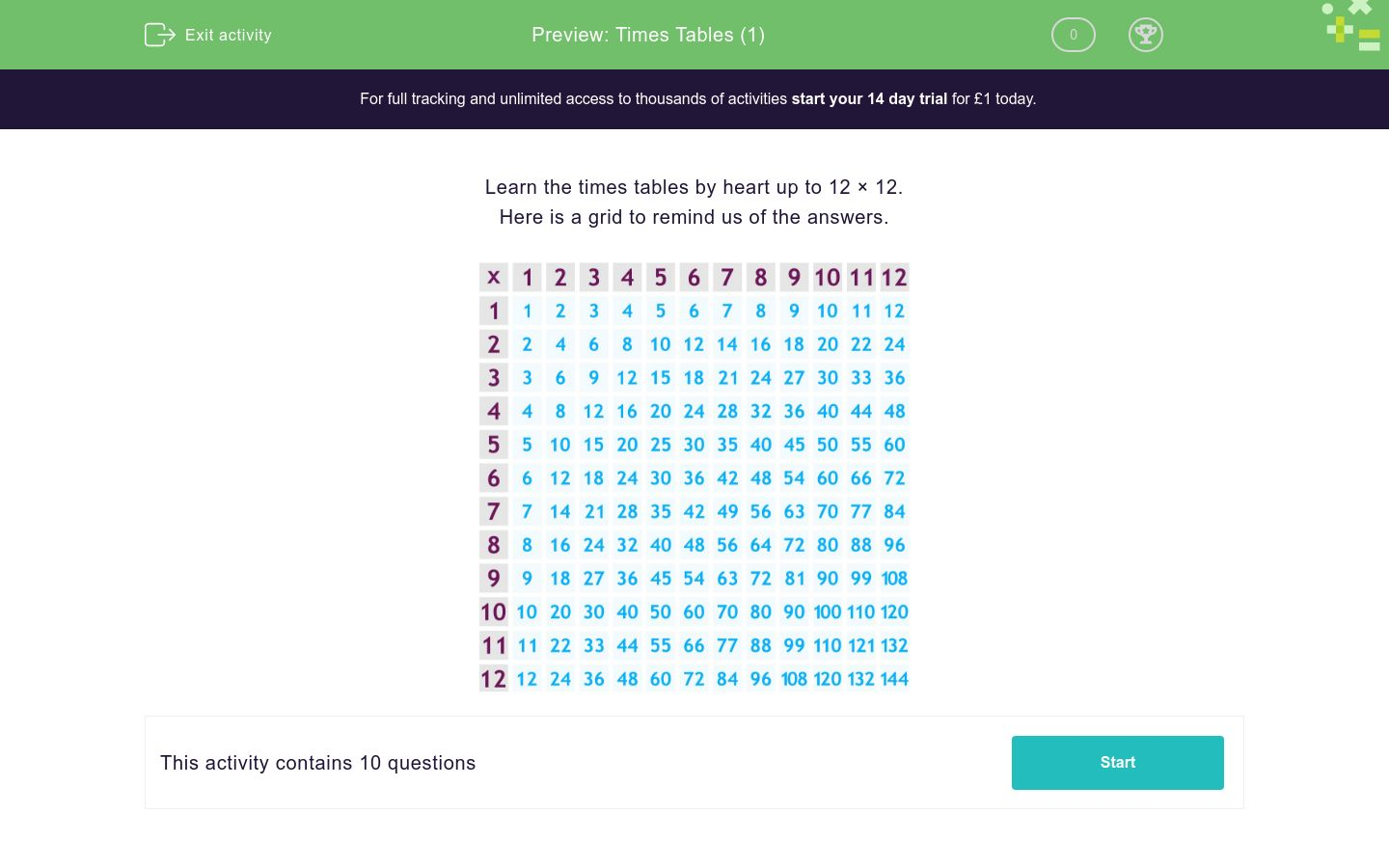# Times Tables (1)

In this worksheet, students must know their tables by heart up to 12. There are 20 questions and no explanations are given.Key stage:  KS 2

Curriculum topic:   Number: Multiplication and Division

Curriculum subtopic:   Multiply/Divide Mentally

Difficulty level:### QUESTION 1 of 10

Learn the times tables by heart up to 12 × 12.

Here is a grid to remind us of the answers.Work out:

5 × 4

Work out:

3 × 5

Work out:

4 × 7

Work out:

2 × 8

Work out:

1 × 12

Work out:

6 × 4

Work out:

5 × 8

Work out:

8 × 4

Work out:

7 × 11

Work out:

6 × 6

• Question 1

Work out:

5 × 4

20
EDDIE SAYS
5 + 5 + 5 + 5 = 20
• Question 2

Work out:

3 × 5

15
EDDIE SAYS
3 + 3 + 3 + 3 + 3 = 15
• Question 3

Work out:

4 × 7

28
EDDIE SAYS
4 + 4 + 4 + 4 + 4 + 4 + 4 = 28
• Question 4

Work out:

2 × 8

16
EDDIE SAYS
Two eight times is 16.
• Question 5

Work out:

1 × 12

12
EDDIE SAYS
Anything multiplied by 1 is itself!
• Question 6

Work out:

6 × 4

24
EDDIE SAYS
6 + 6 + 6 + 6 = 24
• Question 7

Work out:

5 × 8

40
EDDIE SAYS
5 eight times is 40.
• Question 8

Work out:

8 × 4

32
EDDIE SAYS
8 + 8 + 8 + 8 = 32
• Question 9

Work out:

7 × 11

77
EDDIE SAYS
11 lots of 7 is 77.
• Question 10

Work out:

6 × 6

36
EDDIE SAYS
6 + 6 + 6 + 6 + 6 + 6 = 36
---- OR ----